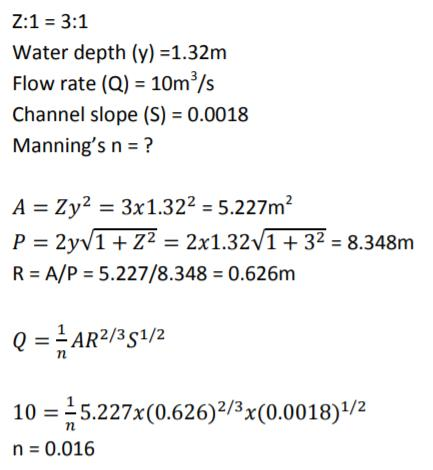Courses

# Open Channel Flow

## 20 Questions MCQ Test Mock Test Series for Civil Engineering (CE) GATE 2020 | Open Channel Flow

Description
This mock test of Open Channel Flow for GATE helps you for every GATE entrance exam. This contains 20 Multiple Choice Questions for GATE Open Channel Flow (mcq) to study with solutions a complete question bank. The solved questions answers in this Open Channel Flow quiz give you a good mix of easy questions and tough questions. GATE students definitely take this Open Channel Flow exercise for a better result in the exam. You can find other Open Channel Flow extra questions, long questions & short questions for GATE on EduRev as well by searching above.
QUESTION: 1

### One kg force is equal to

Solution:

1 kg force= g N where g= acceleration due to gravity = 9.8 m/s2

QUESTION: 2

### A partially open sluice gate discharges water into a rectangular channel. The tail water depth in the channel is 3m and Froude number is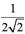. If a free hydraulic jump is to be formed at downstream of the sluice gate at vena contracta of the jet coming out from the sluice gate, the sluice gate opening should be (Coefficient of contraction Cc = 0.9)

Solution: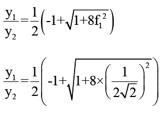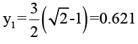Opening the sluice gate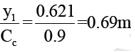QUESTION: 3

### On sag (or valley) curves the available sight distance is determined based on :

Solution:

In valley curves two conditions are required-
Safety Criteria  In which the driver should have adequate light during night.
Comfort Criteria In which the change in centrifugal acceleration is limited upto a certain level.

QUESTION: 4

The sequent depths in a hydraulic jump formed in a 5m wide rectangular channel are 0.2m and 1m. The discharge in the channel is:

Solution: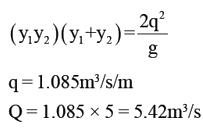QUESTION: 5

Maximum size of a fillet weld for a plate of square edge is

Solution:

The maximum size of a fillet weld is obtained by subtracting 1.5 mm from the thickness of the thinner member to be jointed. This specification limits the size of the fillet weld so that total strength may be developed without overstressing the adjacent metal.

QUESTION: 6

The number of simultaneous equations to be solved in the slope deflection method, is equal to :

Solution:

For every joint a slope deflection equation will be written of the form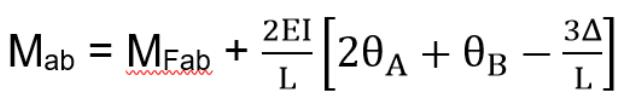Mab = MFab + 2EI/L [2θ_A+θ_B-3Δ/L]
Thus, number of simultaneous equations available will be equal to the number of joints.

QUESTION: 7

A rectangular channel carries a certain flow for which the alternate depths are found to be 3m and 1m. The critical depth (in m) for this flow is:

Solution: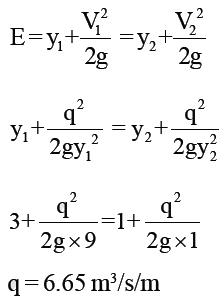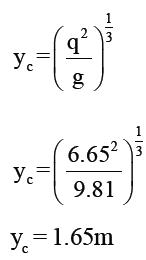QUESTION: 8

In a triangular channel, the critical depth is 2m. The corresponding least possible specific energy is:

Solution: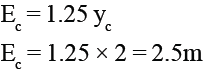QUESTION: 9

If the Froude number of flow in a rectangular channel at a depth of flow of y0 is F0, then what is yc/y0 equal to?

Solution: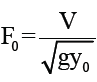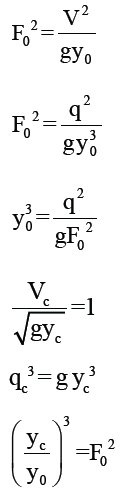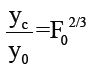QUESTION: 10

A rectangular channel 3m wide carries uniform flow of water at a rate of 7 cumec/sec at a depth of 1.5m. If there is a local rise of 0.5m in the bed, calculate the change in upstream depth of flow?

Solution: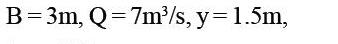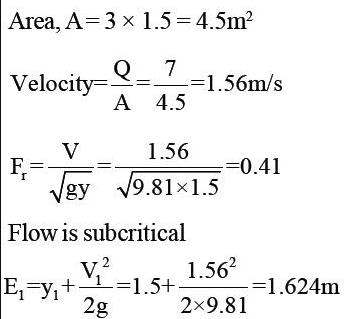As bed level  rises by 0.5m, specific energy at section  2 decreasees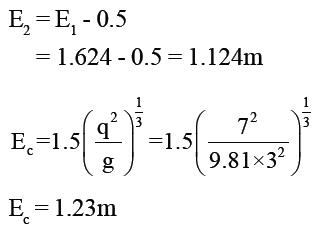Since, E2<Ec hence there is change in upstream depth  condition.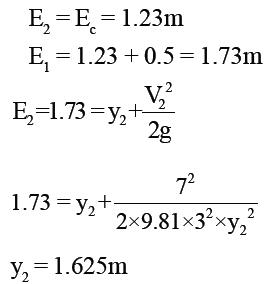Increase in upstream depth
= 1.625 - 1.5
= 0.125m

QUESTION: 11

A wide rectangular channel carries discharge of 6 m3/s per meter width. The channel has a bed slope of 0.009 and Manning's roughness coefficient, n = 0.015. At a certain section of the channel, the flow depth is 1.5m. What gradually varied flow profile exist at this section ?

Solution: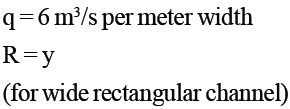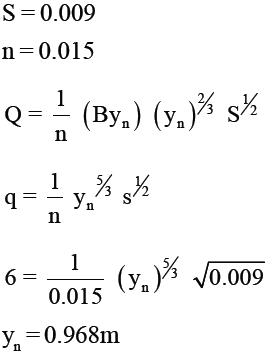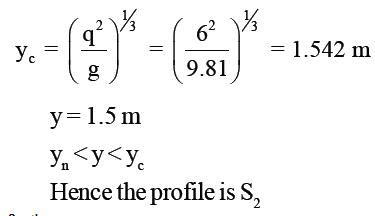QUESTION: 12

In a hydraulic jump occurring in a rectangular channel of 3m width, the discharge is 8 m3/s, and the depth of flow before jump is 0.25m. Find the energy loss in the jump ?

Solution: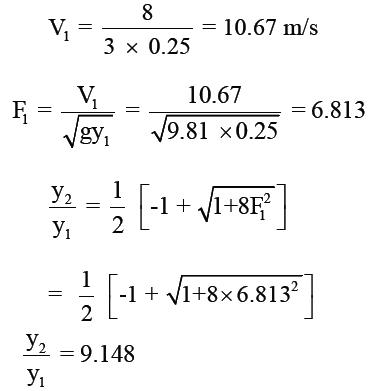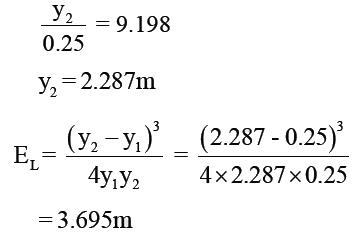QUESTION: 13

The sequent depth ratio in a hydraulic jump formed in a horizontal rectangular channel is 16.48. The Froude number of the super critical stream is :

Solution: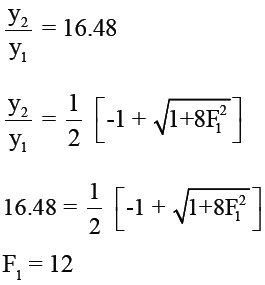QUESTION: 14

A mild slope channel enters a lake with a sudden drop in bed. If the depth of water in the lake measured above the channel bed at its outlet yL is greater than the critical depth, then the depth of flow in the canal at the outlet yd :

Solution:
QUESTION: 15

Water emerges from an ogee spillway with velocity 13.72 m/s and depth = 0.3 m at its toe. The tail water depth required to form a hydraulic jump at the toe is

Solution: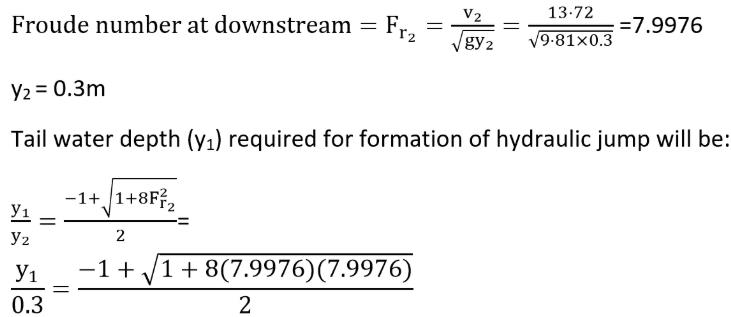y_1 = 3.2464 m

QUESTION: 16

The ratio of unconfined compressive strength of an undisturbed sample of soil to that of a remoulded sample, at the same water content, is known as :

Solution:

Sensitivity is the ratio of unconfined compressive strength of cement of an undisturbed sample to unconfined compressive strength of remoulded sample.
Activity is the ratio of plasticity index to percentage of particles finer than 2 micron.

QUESTION: 17

In a channel the bed slope changes from mild slope to steep slope. The resulting GVF profiles are :

Solution: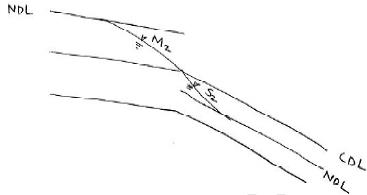Flow profile is (M2,S2)

QUESTION: 18

In a wide river the depth of flow at a section is 3m, S0 = 1 in 5000 and q = 3m3/s per meter width. If the Chezy formula with C = 70 is used, the water surface slope relative to the bed at the section is:

Solution: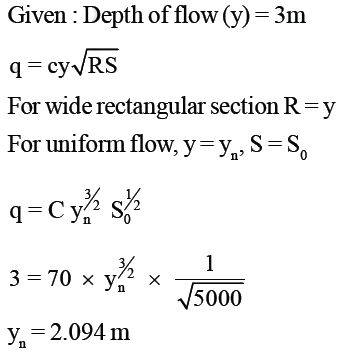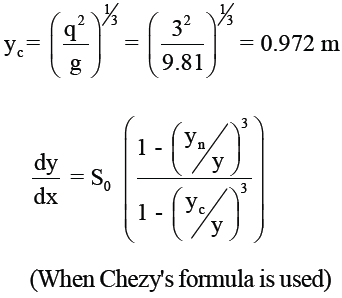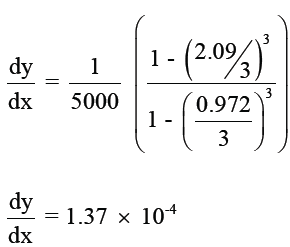QUESTION: 19

At critical depth :

Solution:

At critical depth discharge is maximum for a given specific energy. Also, it can be said as that specific energy is minimum for a given discharge. Also, at critical depth Froude number is equal to 1.

QUESTION: 20

A triangular channel of side slope 3:1 carries water at a flow rate of 10m3 /s. If the depth of the flow is 1.32m and the channel is laid on slope of 0.0018, find Manning’s n.

Solution: